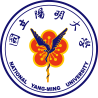# MoreNational Yang Ming University

## Explanation:

\frac{d[mRNAT7]}{dt}=\frac{ [ROSoxyR]^{nROSoxyR} }{ KdROSoxyR^{nROSoxyR}+[ROSoxyR]^{nROSoxyR} }\times{PoPStrxC}\times\frac{N}{V}\times{a}+\frac{ [T7]^{nT7} }{ KdT7^{nT7}+[T7]^{nY7} }\times{PoPST7}\times\frac{N}{V}-KdegmRNA\times[mRNAPDC]

PoPS represents the promoter strength which is measured by the rate of RNApolymerase binding to the starting site of DNA transcription; \frac{ [molecule]^{nmolecule} }{ Kdmolecule^{nmolecule}+[molecule]^{nmolecule} } represents the hill effect of transcription factors (either activators or repressors) to the promoter.

In this equation, the production rate of mRNAT7 is composed of both synthesizing rate and degrading rate. \frac{ [ROSoxyR]^{nROSoxyR} }{ KdROSoxyR^{nROSoxyR}+[ROSoxyR]^{nROSoxyR} }\times{PoPStrxC}\times\frac{N}{V}\times{a} represents how activator ROSoxyR influences promoter trxC on synthesizing mRNAT7 and how thresholds behind the promoter blocks the synthesis of mRNAT7 (the possibility of RNA polymerase to skip thresholds right after trxC promoter is shown in probability);\frac{ [T7]^{nT7} }{ KdT7^{nT7}+[T7]^{nY7} }\times{PoPST7｝\times\frac{N}{V}represents how T7 polymerase influences promoter T7 on synthesizing mRNAT7; -KdegmRNA\times[mRNAT7] represents the natural degrading rate of mRNAT7.

\frac{d[protein of T7 polymerase ]}{dt}=RBS\times{PoPStrxC}\times\frac{N}{V}\times{a}\times{\frac{ [ROSoxyR]^{n1} }{ [Kd]^{n1}+[ROSoxyR]^{n1} }}}+PoPST7\times{T7 effect}\times\frac{N}{V}-{KdegT7}\times{T7}

In this equation, the production rate of T7 polymerase is composed of both synthesizing rate and degrading rate. RBS represents ribosome binding site strength, which is the affinity of ribosome to the starting site of mRNA; PoPS represents the promoter strength and \frac{[molecule]^{nmolecule}}{ Kdmolecule^{nmolecule}+[molecule]^{nmolecule} }, as mentioned above, represents the hill effect of transcription factors to the promoter.

For the section of the equation,\RBS\times{PoPStrxC}\times\frac{N}{V}\times{a}\times{\frac{ [ROSoxyR]^{n1} }{ [Kd]^{n1}+[ROSoxyR]^{n1} }}} represents the synthesizing rate of T7 polymerase under the influence of activator ROSoxyR complex, promoter trxC and terminators downstream; \PoPST7\times{T7 effect}\times\frac{N}{V}, similarly, represents the synthesizing rate of T7polymerase under the influence of T7promoter; \-{KdegT7}\times{T7}represents the natural degrading rate of T7 polymerase.

\frac{d[mRNAPDC]}{dt}=\frac{ [T7]^{nT7} }{ KdT7^{nT7}+[T7]^{nY7} }\times{PoPST7}\times\frac{N}{V}-KdegmRNA\times[mRNAPDC]

In these two equations, the production rate of mRNAPDC and mRNAADH is composed of both synthesizing rate and degrading rate. \frac{ [T7]^{nT7} }{ KdT7^{nT7}+[T7]^{nY7} }\times{PoPST7｝\times\frac{N}{V} represents how T7 polymerase influences promoter T7 on synthesizing mRNAPDC and mRNAADH;\frac{ [T7]^{nT7} }{ KdT7^{nT7}+[T7]^{nY7} } and\frac{ [T7]^{nT7} }{ KdT7^{nT7}+[T7]^{nY7} } represents the natural degrading rate of mRNAPDC and [mRNAADH].

\frac{d[PDC]}{dt}=RBS\times{PoPST7effect}\times\frac{N}{V}-{kdegPDC}\times[PDC}]